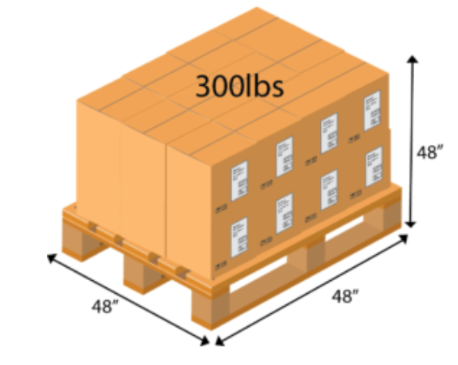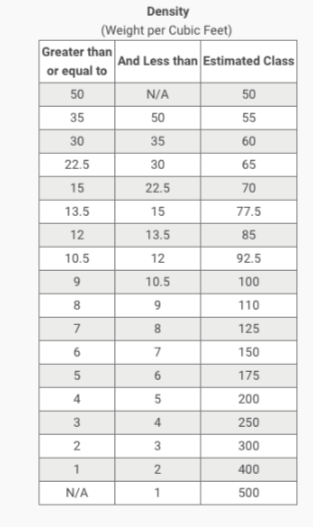# What is density?

Density is a measure of mass per unit of volume.

### Density=Mass/Volume

• The average density of an object equals its total mass divided by its total volume.
• An object made from a comparatively dense material (such as iron) will have less volume than an object of equal mass made from some less dense substance (such as water)

Density is an important component to LTL freight classifications.

A shipment with a lower density typically has a higher freight classification, while a shipment with a higher density typically has a lower freight classification.

### What is dimensional weight?

Dimensional weight is a standard formula used that considers a package’s density when determining charges.

Freight charges are based on the gross weight of the shipment or the dimensional weight of the shipment, whichever is greater.

A large empty box, for example, will be billed according to its L x W x H axis dimensions instead of its weight.

### When do dimensional weight charges apply?

When the actual weight of a package is less than the calculated dimensional weight, carriers charge by the dimensional weight. L x W x H divided by 166 = Dimensional Weight in LBS.# How is freight density calculated?

Let's start with an example shipment: A 40" by 48" pallet is stacked with boxes of products 48" high. The pallet and its contents weigh a total of 425 pounds.

1. Find and note the dimensions and total weight
• Dimensions: Length = 40 in | Width = 48 in | Height = 48 in Total Weight = 425 lbs
2. Volume is calculated by multiplying the dims together, giving a total in cubic inches (volume = LxWxH)
• 40 x 48 x 48 = 92160 cubic inches
3. Convert the volume from cubic inches to cubic feet (1728 cubic inches = 1 cubic foot)
• 92160 / 1728 = 53.33 cubic feet
4. To calculate the density the weight (mass) is divided by the volume (density = m/V)
• 425 / 53.33 = 7.97 lbs per cubic foot
5. Use the density total to determine the freight class (table below)
• Density = 7.97 lbs PCF (per cubic foot)
6. Solution: Class = 125
• Because the density is greater than 7 but less than 8

## Freight Class Reference Chart## Determine LTL Freight Class

Shippers can determine the appropriate Freight Class for a commodity in different ways:

• Use our FreightWise Density Calculator (available in the portal - the calculator icon under the LTL Quote tab and the BOL tab)
• Using the NMFC book available at http://www.nmfta.org/Pages/welcome.aspx
• Using a program such as ClassIT or Fast Class